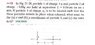# Placing a 3rd charge so there is no net force

• jegues
I'm not sure what you're trying to do.)Exactly. Now revise your equations accordingly. (Check the sign of each term. And somehow you got a numerical value for one of your forces.)(You can also use an equation for F3, if you... I'm not sure what you're trying to do.)f

## Homework Statement

See figure attached for problem statement as well as the figure given.

## The Attempt at a Solution

If a throw a third charge 3 into the mix the forces on each charge should be as follows:

$$\vec{F_{1}} = \vec{F_{13}} + \vec{F_{12}}$$

$$\vec{F_{2}} = \vec{F_{21}} + \vec{F_{23}}$$

$$\vec{F_{3}} = \vec{F_{31}} + \vec{F_{32}}$$

Is this correct?

Is it also correct to say that,

$$\vec{F_{13}} = - \vec{F_{31}}$$

Am I taking the right route to solving the problem?

#### Attachments

•CH21-P19.JPG
36.3 KB · Views: 521
If a throw a third charge 3 into the mix the forces on each charge should be as follows:

$$\vec{F_{1}} = \vec{F_{13}} + \vec{F_{12}}$$

$$\vec{F_{2}} = \vec{F_{21}} + \vec{F_{23}}$$

$$\vec{F_{3}} = \vec{F_{31}} + \vec{F_{32}}$$

Is this correct?
Sure.

Is it also correct to say that,

$$\vec{F_{13}} = - \vec{F_{31}}$$
Yes.

Am I taking the right route to solving the problem?
So far, so good. Hint: In what region of the x-axis must the third charge be placed?

Sure.

Yes.

So far, so good. Hint: In what region of the x-axis must the third charge be placed?

Well I know that the resulting forces on all three charges should be 0 so looking at F1 and F2,

$$\vec{F_{1}} = \frac{k|q_{3}|}{x^{2}} \hat{i} - 4.44*10^{12} \hat{i} = 0$$

and

$$\vec{F_{2}} = \frac{4k|q_{3}|}{(x-0.09)^{2}} \hat{i} + 4.44*10^{12} \hat{i} = 0$$

I have two equations with two unknowns so I should be able to solve it.

Is there an easier way or did I make a mistake?

Note that the sign of the force from a charge depends on which side of the charge you are on. See my hint in my last post.

Note that the sign of the force from a charge depends on which side of the charge you are on. See my hint in my last post.

Ah, I didn't know that!

So then the third charge must be placed somewhere inbetween the the two charges 1 and 2, correct?

But how do I find it's x coordinate?

So then the third charge must be placed somewhere inbetween the the two charges 1 and 2, correct?
Exactly!

But how do I find it's x coordinate?
Set up your force equations as before. Now that you know what region to worry about--and thus what side of each charge you're on--you should be able to do it.

Exactly!

Set up your force equations as before. Now that you know what region to worry about--and thus what side of each charge you're on--you should be able to do it.

I don't understand how this changes my equations I had previously?

What must be the sign of the third charge? How does that affect the signs of the forces?

What must be the sign of the third charge? How does that affect the signs of the forces?

It has to be positive, it will reserve the directions in which the forces act.

EDIT: Well actually, it could be either positive or negative, you'd just have to move the charge accordingly so that the forces cancel out.

It has to be positive, it will reserve the directions in which the forces act.
If all three charges are positive, how can q1 or q3 be in equilibrium?

If all three charges are positive, how can q1 or q3 be in equilibrium?

I'm overwhelmed with confusion as to what I did right and what I did wrong in this problem, or even what I understood correctly and what I misunderstood completely.

Are we still working from the 2 equations I gave previously?

Are we still working from the 2 equations I gave previously?
You'll have to correct those equations. First step: answer my question about the sign of q3. (Don't plug in any numbers until the final step, if you can help it.)

You'll have to correct those equations. First step: answer my question about the sign of q3. (Don't plug in any numbers until the final step, if you can help it.)

Okay so we have 2 positive charges sitting on the x-axis, we have to decide where to place a third charge, and what sign it should be.

We figured out that the 3rd charge must be placed inbetween the two original charges from my 2 equations (they still need to be fixed?).

So now we have to figure out whether this charge is going to be positively charged or negatively charged.

If all three charges are positive, how can q1 or q3 be in equilibrium?

Well the two original charges are positive, so they are repelling each other, so to counteract this repulsion I MUST place a negative charge somewhere inbetween the two.

What's next Doc?

Well the two original charges are positive, so they are repelling each other, so to counteract this repulsion I MUST place a negative charge somewhere inbetween the two.
Exactly. Now revise your equations accordingly. (Check the sign of each term. And somehow you got a numerical value for one of your forces.)

(You can also use an equation for F3, if you like.)

Exactly. Now revise your equations accordingly. (Check the sign of each term. And somehow you got a numerical value for one of your forces.)

(You can also use an equation for F3, if you like.)

Thank you! With this knowledge I was able to obtain the correct answer!

You are most welcome!